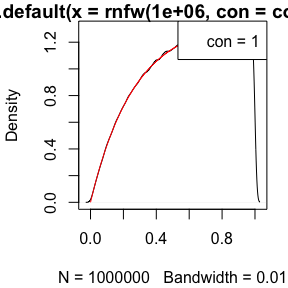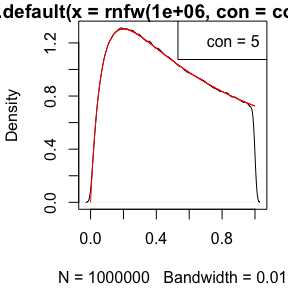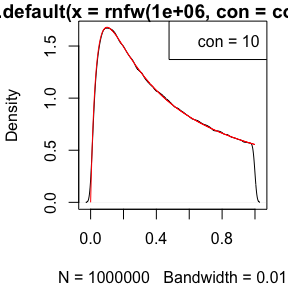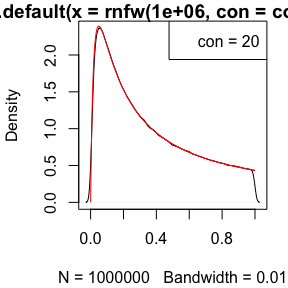NFW Distribution

QDF Proof

This is a compact version of the paper arXiv 1805.09550.

First we define the NFW PDF for any input scale radius $$q=R/R_{vir}$$

$d(q,c) = \frac{c^2}{(c q + 1)^2 \left(\frac{1}{c + 1} + \ln(c + 1) - 1\right)}$

We define the un-normalised integral up to a normalised radius $$q$$ as

$p'(q,c) = \ln(1 + c q)-\frac{c q}{1 + c q}.$

We can define $$p$$, the correctly normalised probability (where $$p(q=1,c)=1$$ with a domain [0,1]) as

$p(q,c) = \frac{p'(q,c)}{p'(1,c)} \Rightarrow p'(q,c)=p(q,c) p'(1,c)$

Using the un-normalised variant $$p'$$ we can state that

$p'+1 = \ln(1 + c q) - \frac{c q}{1 + c q} + \frac{1 + c q}{1 + c q} = \ln(1 + c q) + \frac{1}{1 + c q}.$

Taking exponents and setting equal to $$y$$ we get

$y = e^{p'+1} = (1 + c q) e^{1/(1 + c q)}.$

We can the define $$x$$ such that

$x = 1 + c q, \\ y = x e^{1/x}.$

Here we can use the Lambert W function to solve for $$x$$, since it generically solves for relations like y = x e^{x} (where $$x = W_0(y)$$)). The exponent has a $$1/x$$ term, which modifies that solution to the slightly less elegant

$x = -\frac{1}{W_0(-1/y)} = -\frac{1}{W_0(-1/e^{(p'+1)})}, \\ \text{sub for } q = \frac{x - 1}{c}, \\ q = -\frac{1}{c}\left(\frac{1}{W_0(-1/e^{(p'+1)})}+1\right).$

Given the above, this opens up an analytic route for generating exact random samples of the NFW for any $$c$$ (where we must be careful to scale such that $$p'(q,c)=p(q,c) p'(1,c)$$) via,

$r([0,1]; c) = q(p=U[0,1]; c).$

Some Example Usage

Both the PDF (dnfw) integrated up to x, and CDF at q (pnfw) should be the same (0.373, 0.562, 0.644, 0.712):

for(con in c(1,5,10,20)){
print(integrate(dnfw, lower=0, upper=0.5, con=con)\$value)
print(pnfw(0.5, con=con))
}
##  0.373455
##  0.373455
##  0.5618349
##  0.5618349
##  0.6437556
##  0.6437556
##  0.7116174
##  0.7116174

The qnfw should invert the pnfw, returning the input vector (1:9)/10:

for(con in c(1,5,10,20)){
print(qnfw(p=pnfw(q=(1:9)/10,con=con), con=con))
}
##  0.1 0.2 0.3 0.4 0.5 0.6 0.7 0.8 0.9
##  0.1 0.2 0.3 0.4 0.5 0.6 0.7 0.8 0.9
##  0.1 0.2 0.3 0.4 0.5 0.6 0.7 0.8 0.9
##  0.1 0.2 0.3 0.4 0.5 0.6 0.7 0.8 0.9

The sampling from rnfw should recreate the expected PDF from dnfw:

for(con in c(1,5,10,20)){
par(mar=c(4.1,4.1,1.1,1.1))
plot(density(rnfw(1e6,con=con), bw=0.01))
lines(seq(0,1,len=1e3), dnfw(seq(0,1,len=1e3),con=con),col='red')
legend('topright',legend=paste('con =',con))
}Happy sampling!